Скачать презентацию database-normalisation-example

database-normalisation-example.ppt

• Размер: 517 Кб
• Количество слайдов: 35

## Описание презентации Презентация database-normalisation-example по слайдамA Normalisation Example Based on work by Robert Timmer-Arends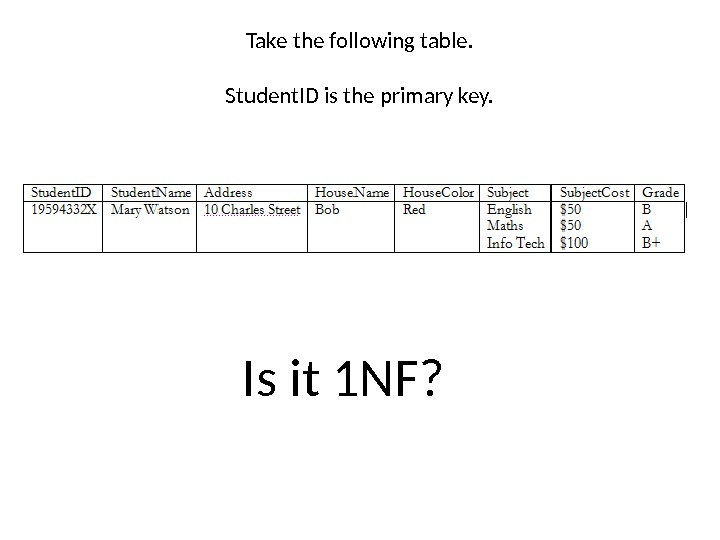Take the following table. Student. ID is the primary key. Is it 1 NF?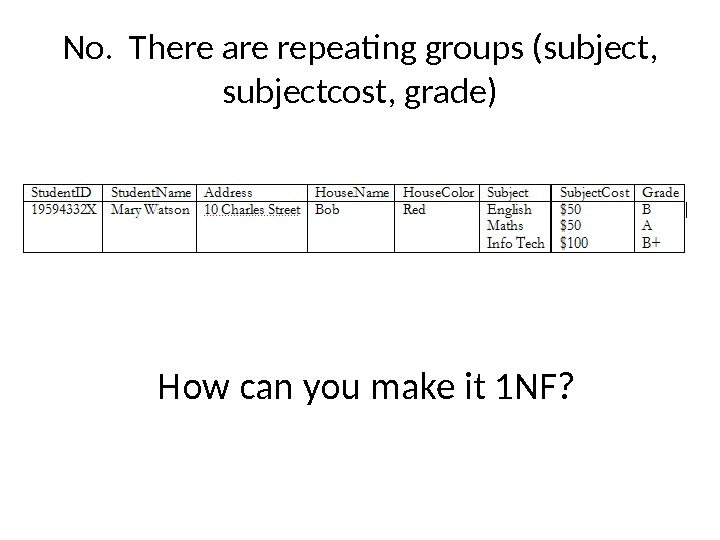No. There are repeating groups (subject, subjectcost, grade) How can you make it 1 NF?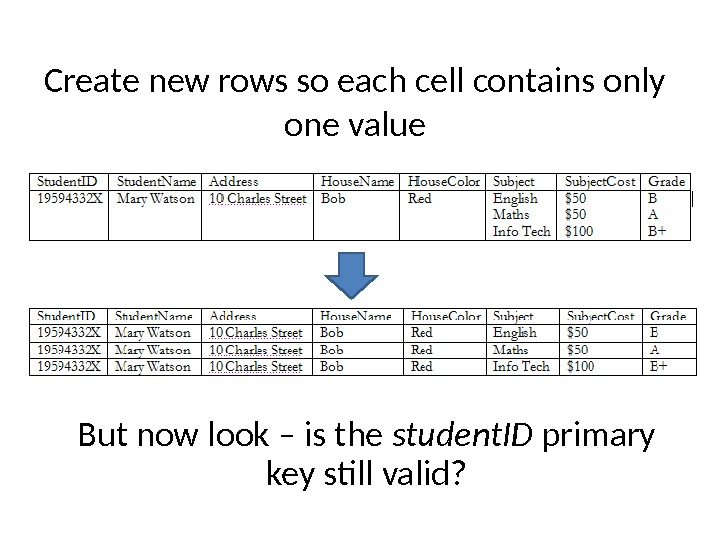Create new rows so each cell contains only one value But now look – is the student. ID primary key still valid?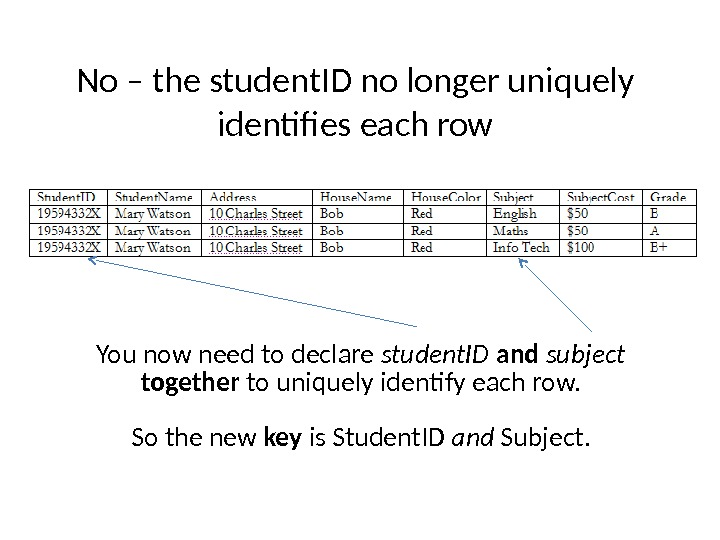No – the student. ID no longer uniquely identifies each row You now need to declare student. ID and subject together to uniquely identify each row. So the new key is Student. ID and Subject.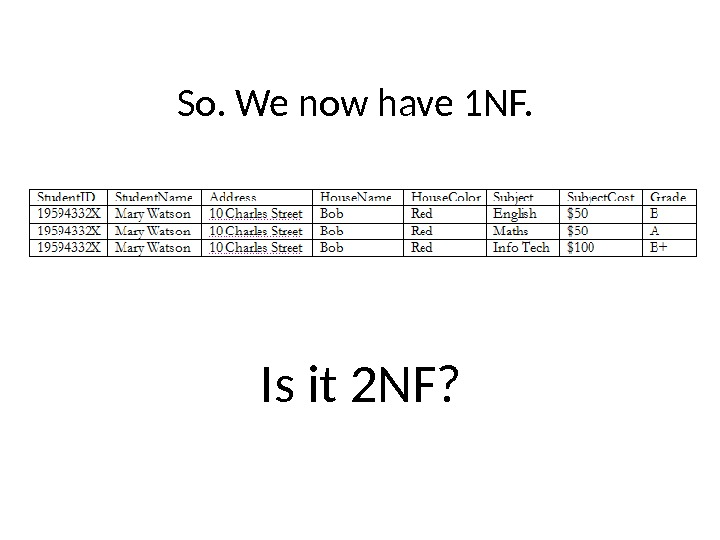So. We now have 1 NF. Is it 2 NF?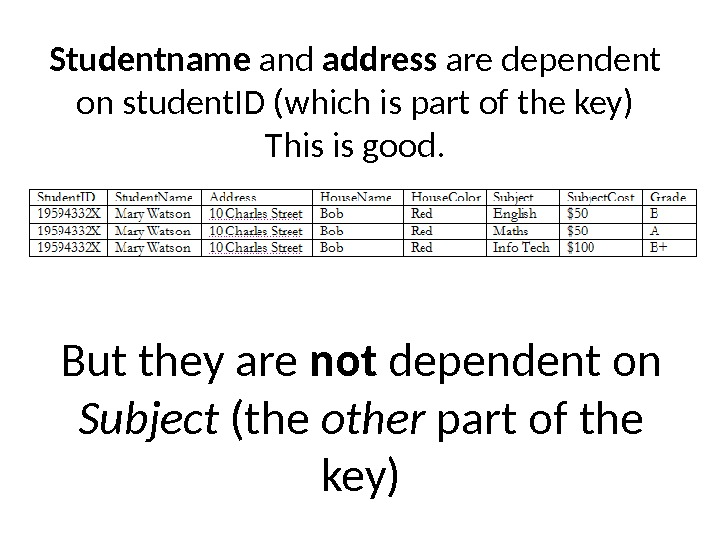Studentname and address are dependent on student. ID (which is part of the key) This is good. But they are not dependent on Subject (the other part of the key)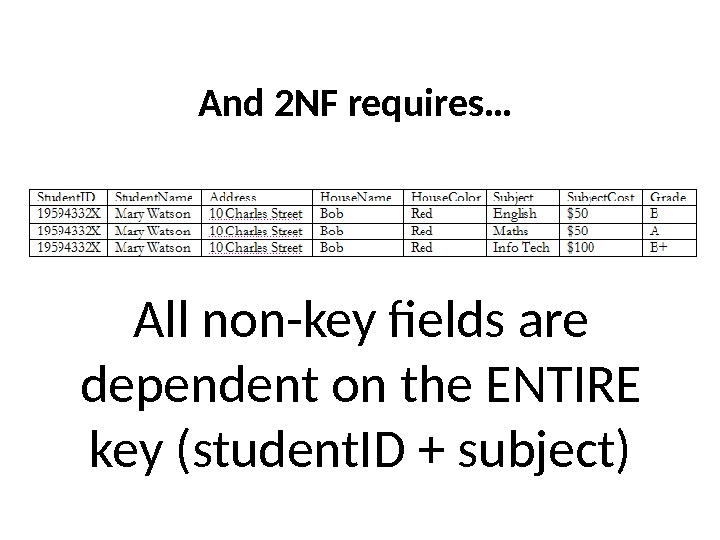And 2 NF requires… All non-key fields are dependent on the ENTIRE key (student. ID + subject)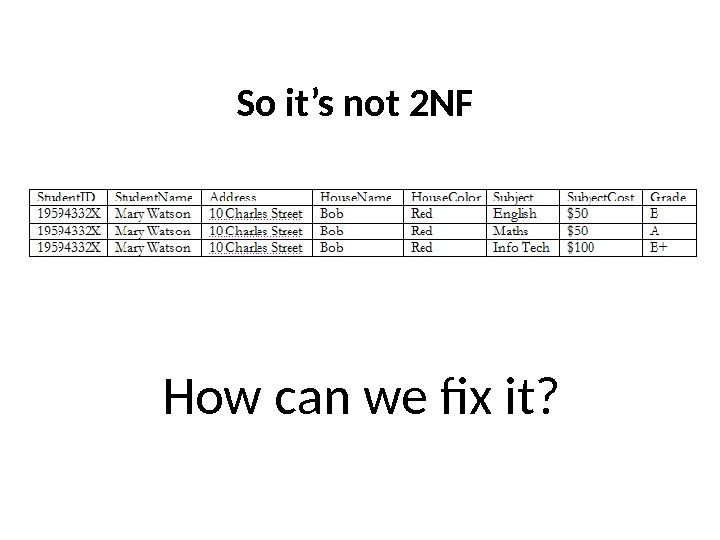So it’s not 2 NF How can we fix it?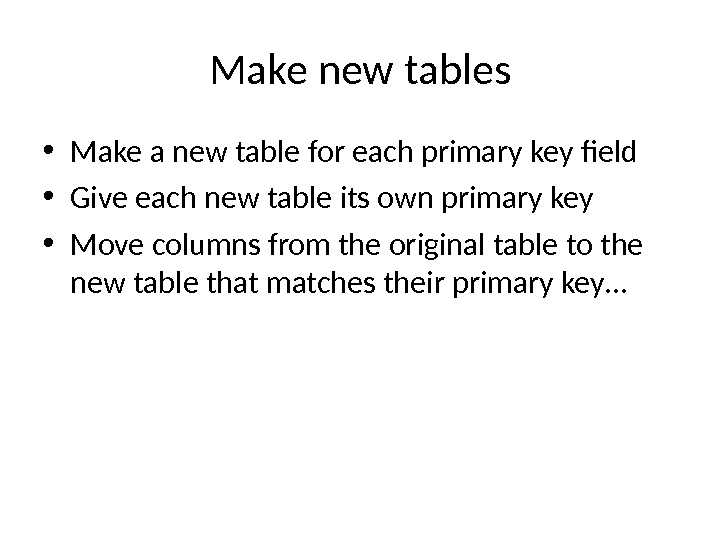Make new tables • Make a new table for each primary key field • Give each new table its own primary key • Move columns from the original table to the new table that matches their primary key…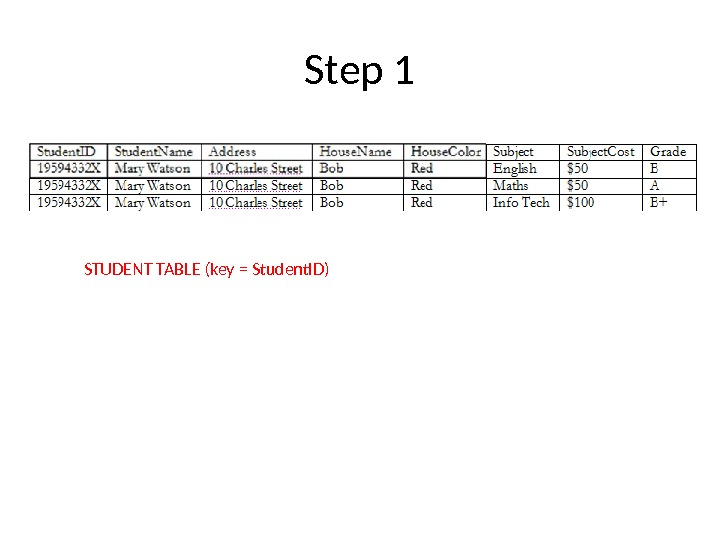Step 1 STUDENT TABLE (key = Student. ID)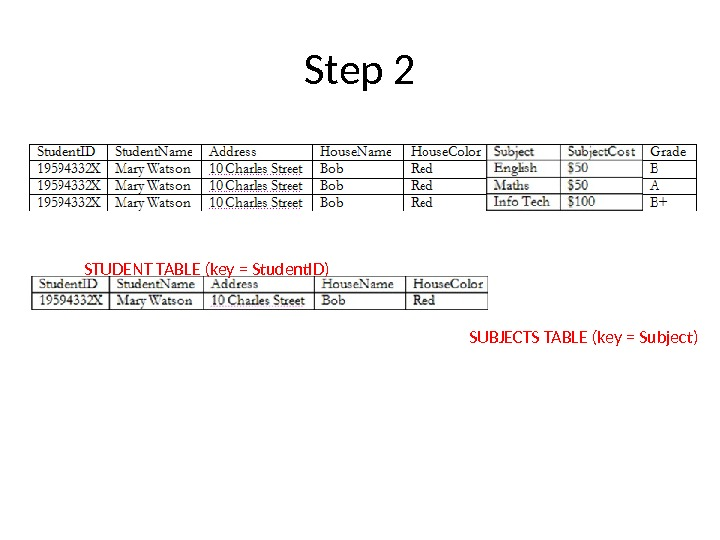Step 2 STUDENT TABLE (key = Student. ID) SUBJECTS TABLE (key = Subject)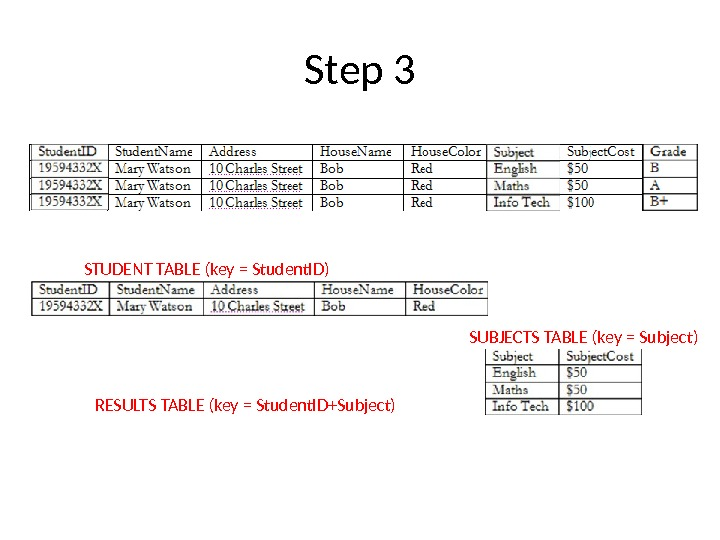Step 3 STUDENT TABLE (key = Student. ID) SUBJECTS TABLE (key = Subject) RESULTS TABLE (key = Student. ID+Subject)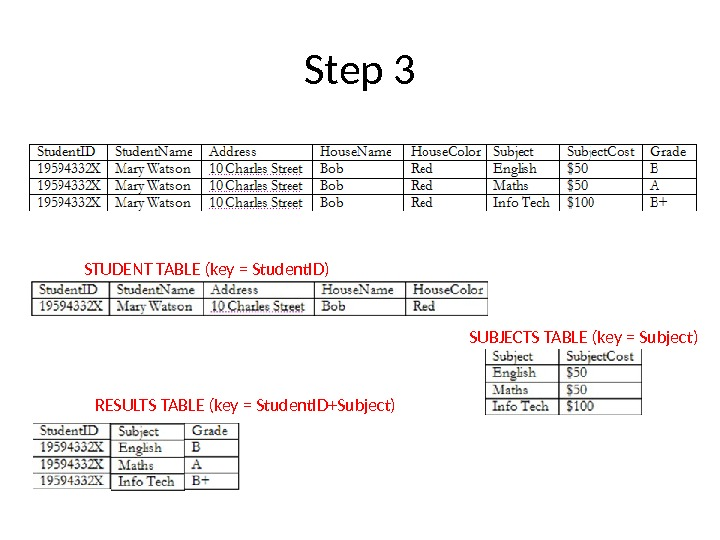Step 3 STUDENT TABLE (key = Student. ID) SUBJECTS TABLE (key = Subject) RESULTS TABLE (key = Student. ID+Subject)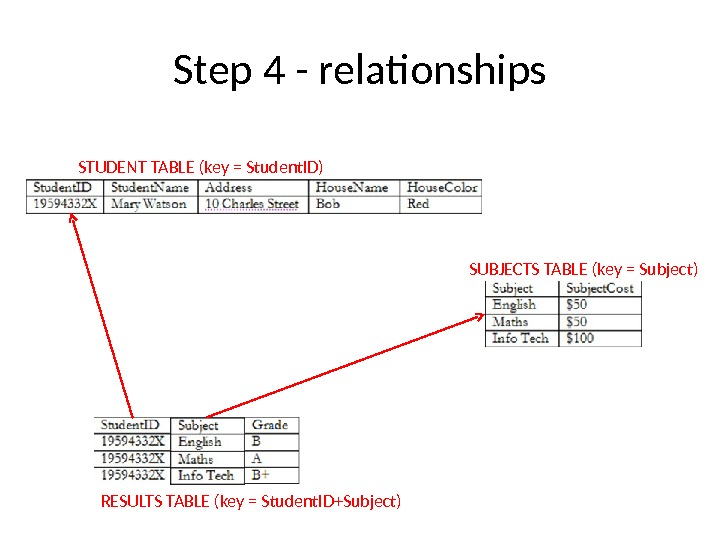Step 4 — relationships STUDENT TABLE (key = Student. ID) SUBJECTS TABLE (key = Subject) RESULTS TABLE (key = Student. ID+Subject)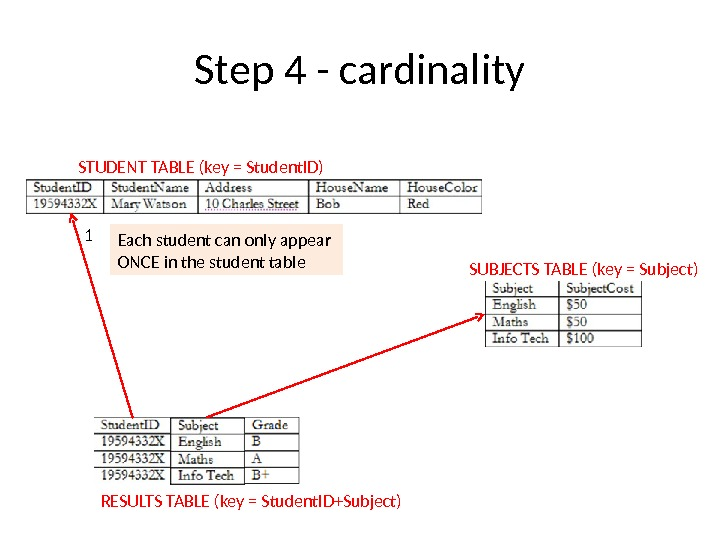Step 4 — cardinality STUDENT TABLE (key = Student. ID) SUBJECTS TABLE (key = Subject) RESULTS TABLE (key = Student. ID+Subject)1 Each student can only appear ONCE in the student table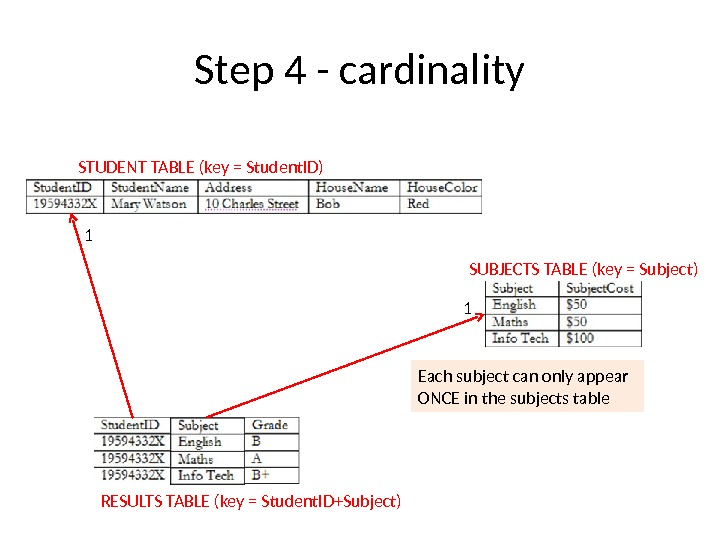Step 4 — cardinality STUDENT TABLE (key = Student. ID) SUBJECTS TABLE (key = Subject) RESULTS TABLE (key = Student. ID+Subject)1 1 Each subject can only appear ONCE in the subjects table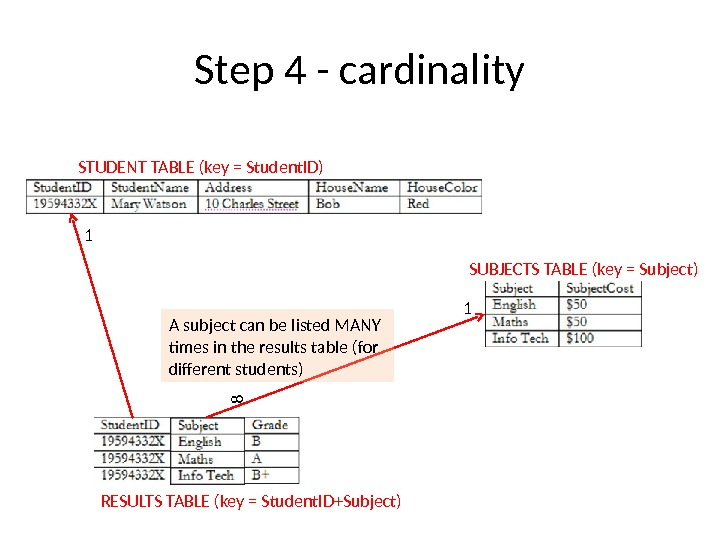Step 4 — cardinality STUDENT TABLE (key = Student. ID) SUBJECTS TABLE (key = Subject) RESULTS TABLE (key = Student. ID+Subject)1 18 A subject can be listed MANY times in the results table (for different students)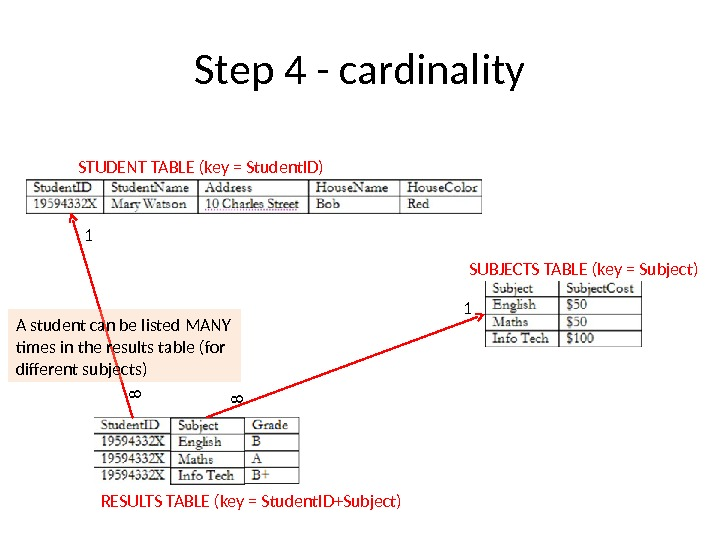Step 4 — cardinality STUDENT TABLE (key = Student. ID) SUBJECTS TABLE (key = Subject) RESULTS TABLE (key = Student. ID+Subject)1 188 A student can be listed MANY times in the results table (for different subjects)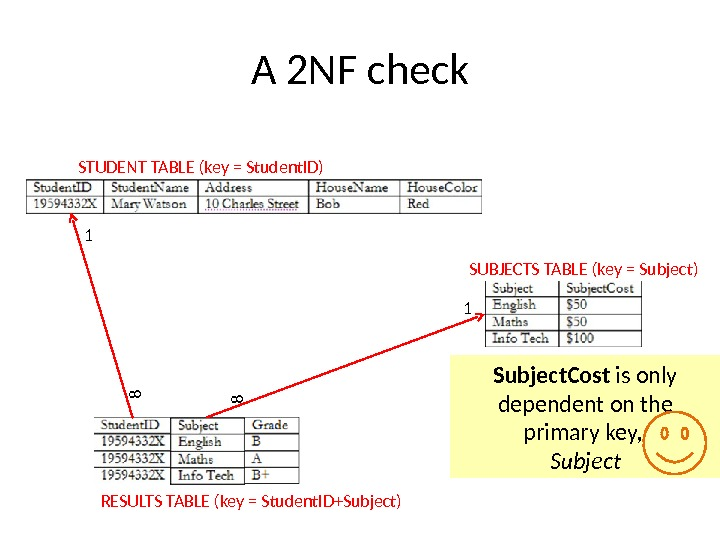A 2 NF check STUDENT TABLE (key = Student. ID) SUBJECTS TABLE (key = Subject) RESULTS TABLE (key = Student. ID+Subject)1 188 Subject. Cost is only dependent on the primary key, Subject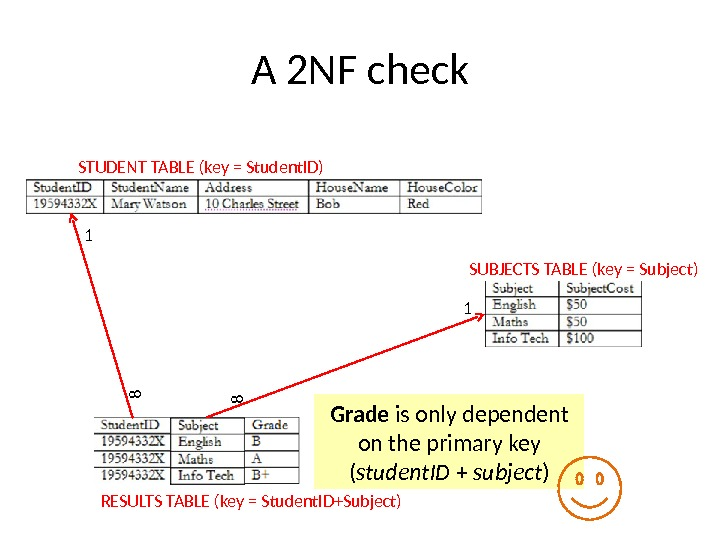A 2 NF check STUDENT TABLE (key = Student. ID) SUBJECTS TABLE (key = Subject) RESULTS TABLE (key = Student. ID+Subject)1 188 Grade is only dependent on the primary key ( student. ID + subject )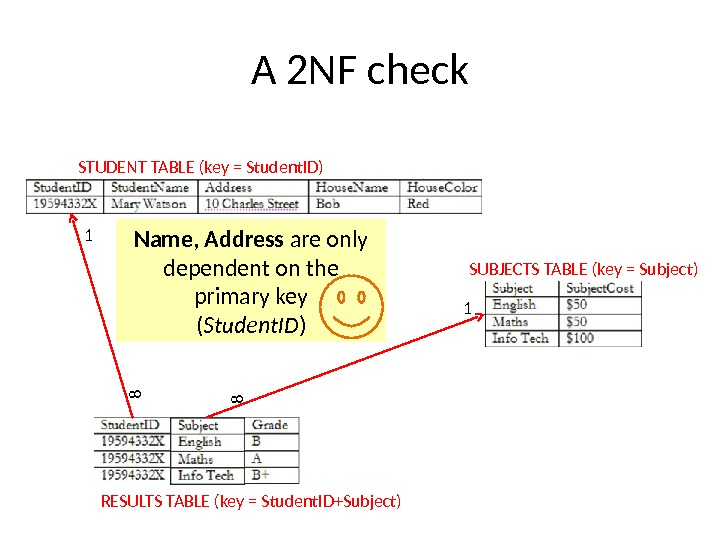A 2 NF check STUDENT TABLE (key = Student. ID) SUBJECTS TABLE (key = Subject) RESULTS TABLE (key = Student. ID+Subject)1 188 Name, Address are only dependent on the primary key ( Student. ID )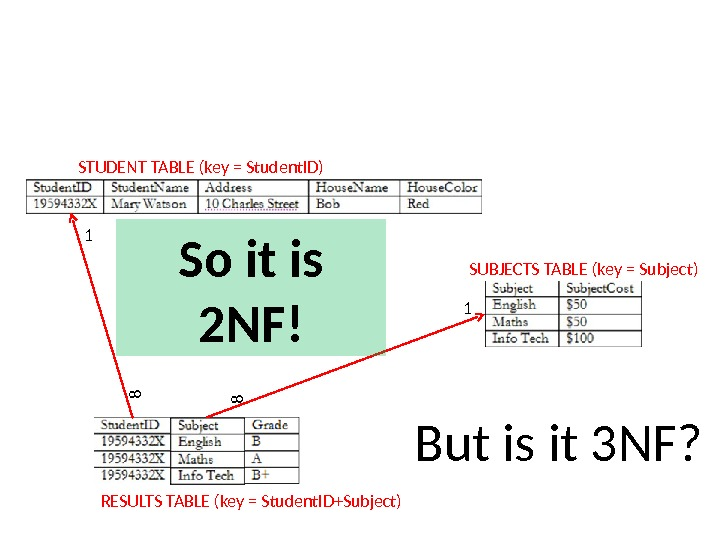But is it 3 NF? STUDENT TABLE (key = Student. ID) SUBJECTS TABLE (key = Subject) RESULTS TABLE (key = Student. ID+Subject)1 188 So it is 2 NF!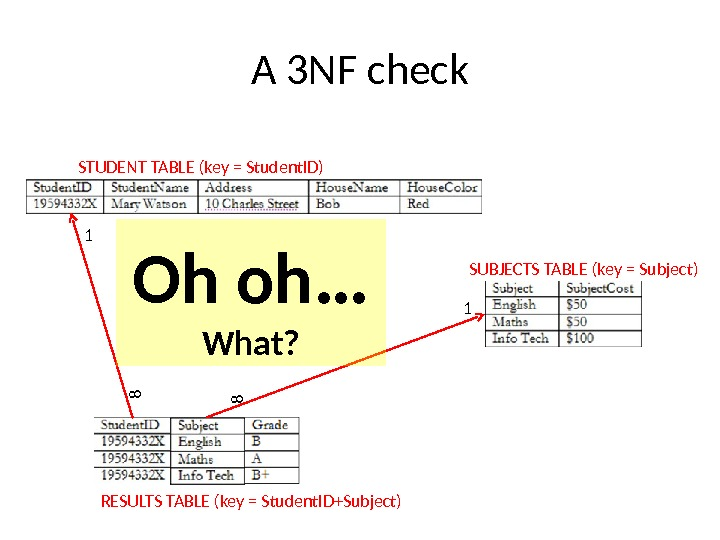A 3 NF check STUDENT TABLE (key = Student. ID) SUBJECTS TABLE (key = Subject) RESULTS TABLE (key = Student. ID+Subject)1 188 Oh oh … What?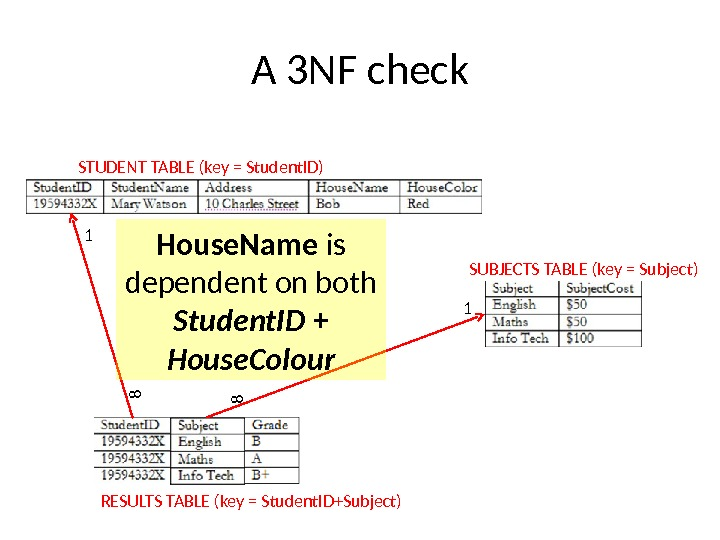A 3 NF check STUDENT TABLE (key = Student. ID) SUBJECTS TABLE (key = Subject) RESULTS TABLE (key = Student. ID+Subject)1 188 House. Name is dependent on both Student. ID + House. Colour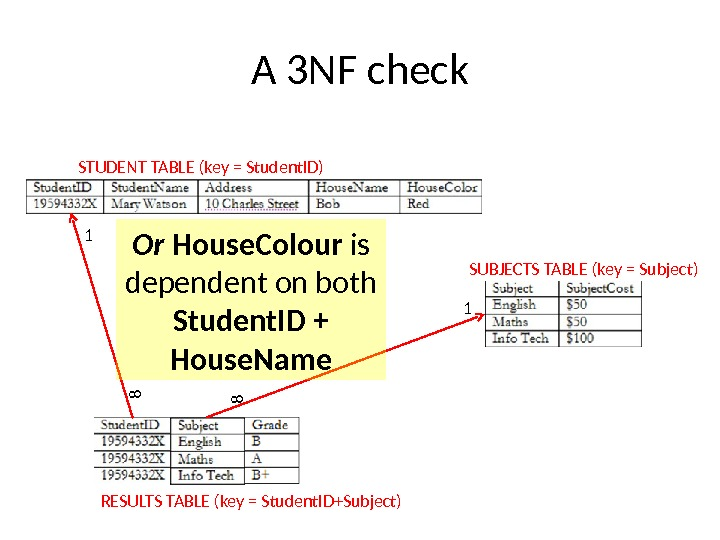A 3 NF check STUDENT TABLE (key = Student. ID) SUBJECTS TABLE (key = Subject) RESULTS TABLE (key = Student. ID+Subject)1 188 Or House. Colour is dependent on both Student. ID + House. Name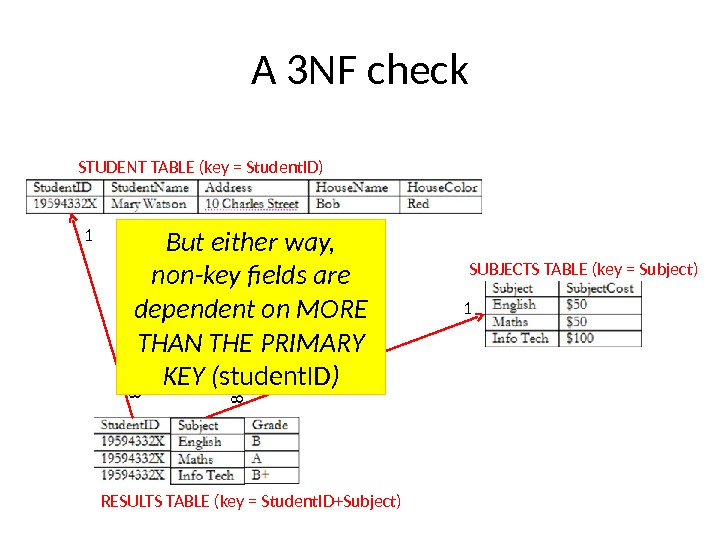A 3 NF check STUDENT TABLE (key = Student. ID) SUBJECTS TABLE (key = Subject) RESULTS TABLE (key = Student. ID+Subject)1 188 But either way, non-key fields are dependent on MORE THAN THE PRIMARY KEY ( student. ID )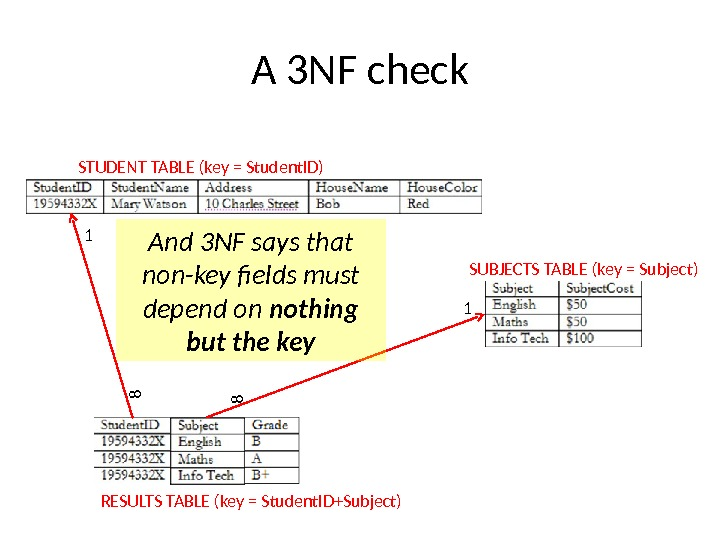A 3 NF check STUDENT TABLE (key = Student. ID) SUBJECTS TABLE (key = Subject) RESULTS TABLE (key = Student. ID+Subject)1 188 And 3 NF says that non-key fields must depend on nothing but the key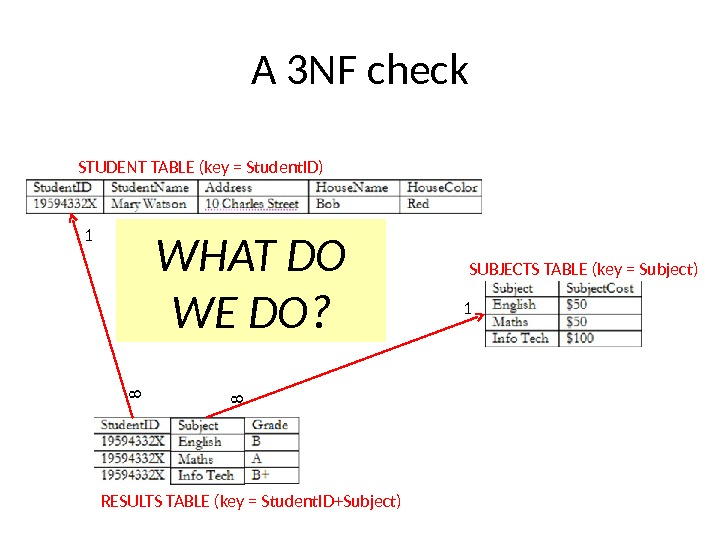A 3 NF check STUDENT TABLE (key = Student. ID) SUBJECTS TABLE (key = Subject) RESULTS TABLE (key = Student. ID+Subject)1 188 WHAT DO WE DO?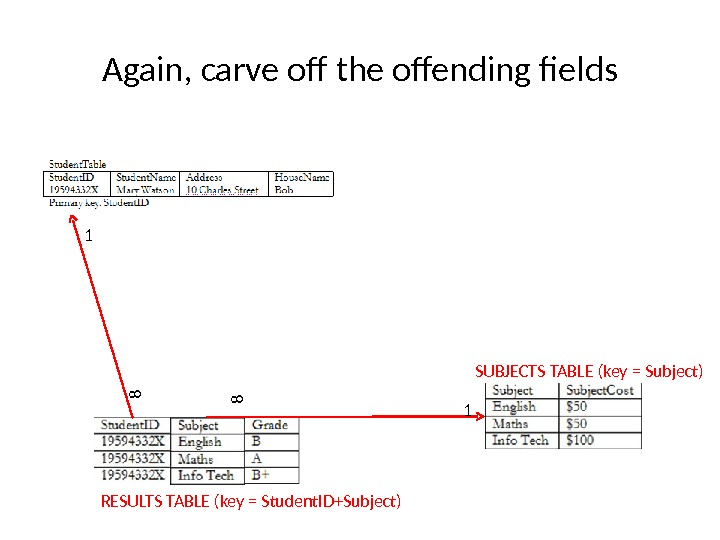Again, carve off the offending fields SUBJECTS TABLE (key = Subject) RESULTS TABLE (key = Student. ID+Subject)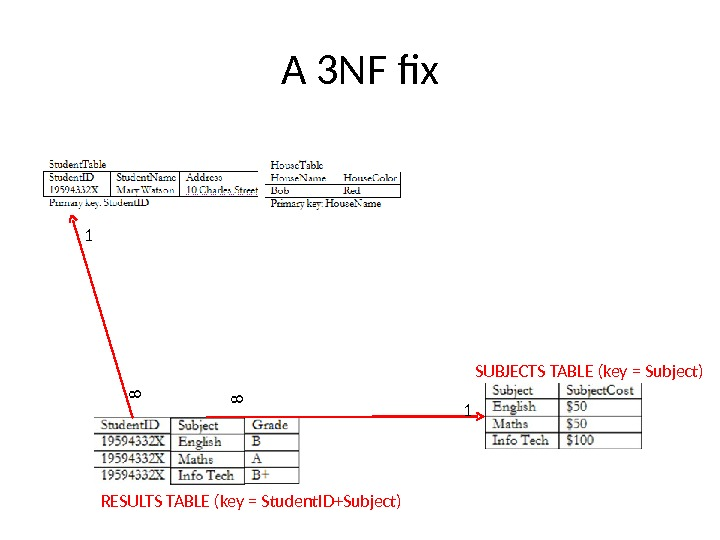A 3 NF fix SUBJECTS TABLE (key = Subject) RESULTS TABLE (key = Student. ID+Subject)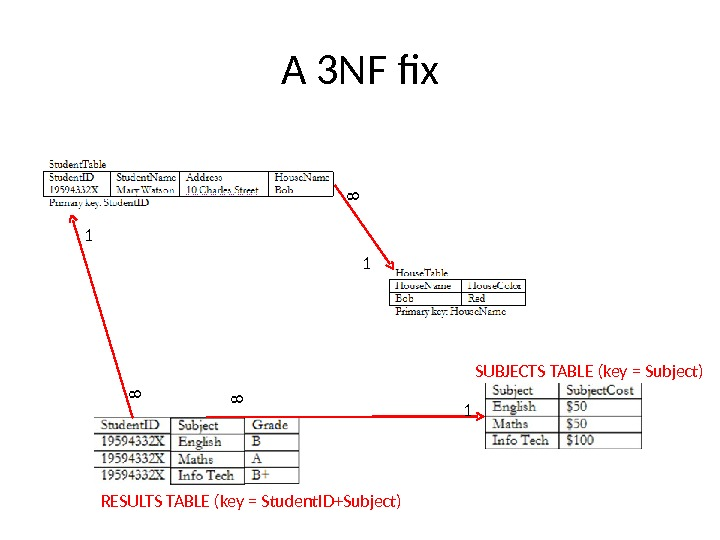A 3 NF fix SUBJECTS TABLE (key = Subject) RESULTS TABLE (key = Student. ID+Subject)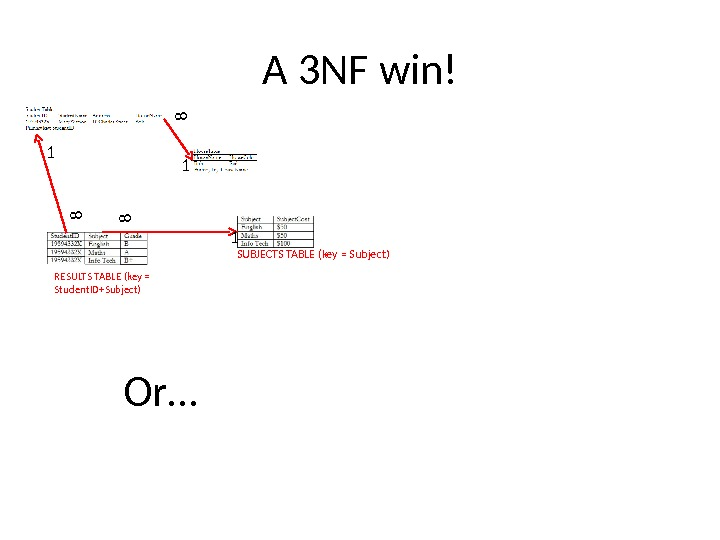A 3 NF win! SUBJECTS TABLE (key = Subject) RESULTS TABLE (key = Student. ID+Subject)1 1881 8 Or…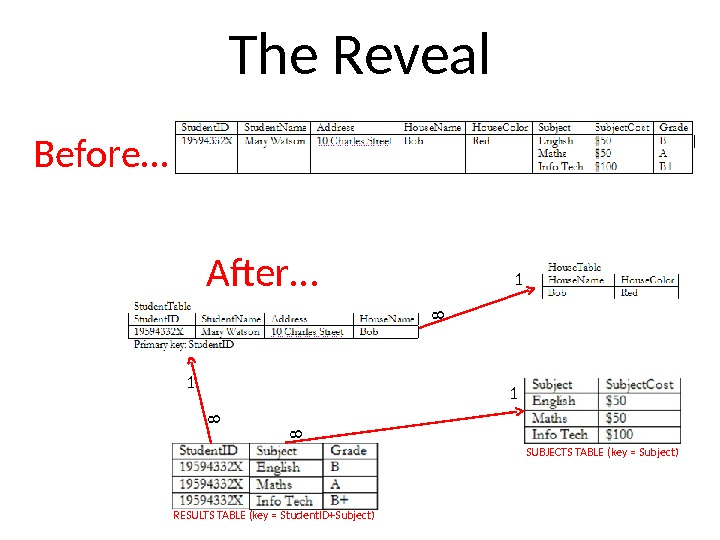The Reveal Before… After… RESULTS TABLE (key = Student. ID+Subject) 1 18 8 1 8 SUBJECTS TABLE (key = Subject)The end • Thanks to Robert Timmer-Arends for the scenario and staging of the normalisation

Зарегистрируйтесь, чтобы просмотреть полный документ!
РЕГИСТРАЦИЯ